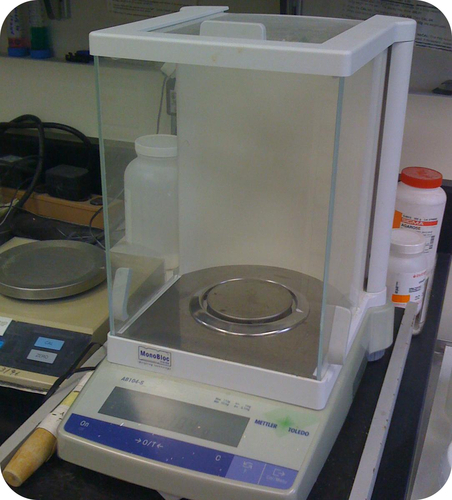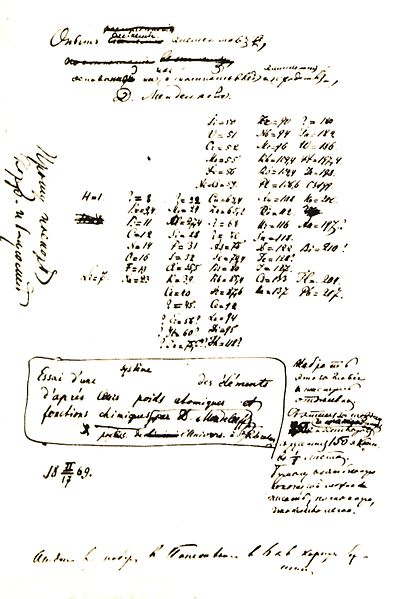## Mass Number

### Learning Objectives

• Define mass number.
• Calculate the mass number when given number of protons and neutrons.
• Calculate number of neutrons when given atomic number.

### How can you determine the mass of a chemical?

Often a student will need to weigh out a chemical for an experiment.  If he or she uses a watch glass (a small, round piece that will hold the solid chemical), the weight of the watch glass must be determined first.  Then the solid is added to the glass and the weight of the glass plus the solid is measured.  The balance reading will be the total of the glass plus the chemical.## History of Atomic Weight DeterminationsAs a part of his research on atoms, John Dalton determined a number of atomic weights of elements in the early 1800s.  Atomic weights were the basis for the periodic table that Mendeleev developed.  Originally all atomic weights were based on a comparison to hydrogen, which has an atomic weight of one.  After the discovery of the proton, scientists assumed that the weight of an atom was essentially that of the protons—electrons were known to contribute almost nothing to the atomic weight of the element.

This approach worked until we learned how to determine the number of protons in an element.  We then saw that the atomic weight for an element was often twice the number of protons (or more).  The discovery of the neutron provided the missing part of the picture.  The atomic mass now known to be the sum of the protons and neutrons in the nucleus.

### Mass Number

Rutherford showed that the vast majority of the mass of an atom is concentrated in its nucleus, which is composed of protons and neutrons. The mass number is defined as the total number of protons and neutrons in an atom. Consider Table below which shows data from the first six elements of the periodic table.

 Name Symbol Atomic Number Protons Neutrons Electrons Mass Number Hydrogen H 1 1 0 1 1 Helium He 2 2 2 2 4 Lithium Li 3 3 4 3 7 Beryllium Be 4 4 5 4 9 Boron B 5 5 6 5 11 Carbon C 6 6 6 6 12

Consider the element helium. Its atomic number is 2, so it has two protons in its nucleus. Its nucleus also contains two neutrons. Since 2 + 2 = 4, we know that the mass number of the helium atom is 4. Finally, the helium atom also contains two electrons since the number of electrons must equal the number of protons. This example may lead you to believe that atoms have the same number of protons and neutrons, but further examination of the Table above will show that this is not the case. Lithium, for example has three protons and four neutrons, leaving it with a mass number of 7.

Knowing the mass number and the atomic number of an atom allows you to determine the number of neutrons present in that atom by subtraction.

Number of neutrons = mass number − atomic number

Atoms of the element chromium (Cr) have an atomic number of 24 and a mass number of 52. How many neutrons are in the nucleus of a chromium atom? To determine this, you would subtract as shown:

52 − 24 = 28 neutrons in a chromium atom

The composition of any atom can be illustrated with a shorthand notation using the atomic number and the mass number. Both are written before the chemical symbol, with the mass number written as a superscript and the atomic number written as a subscript. The chromium atom discussed above would be written as $^{52}_{24}\text{Cr}$.

Another way to refer to a specific atom is to write the mass number of the atom after the name, separated by a hyphen. The above atom would be written as chromium-52.

### Summary

• The mass number is defined as the total number of protons and neutrons in an atom.
• The number of neutrons = mass number − atomic number.

### Practice

http://education.jlab.org/qa/pen_number.html

1. What data in the periodic table tells you the number of protons in an atom?
2. How do you determine the number of neutrons in an atom?
3. What is the mass number for an atom?

### Review

1. Who first determined atomic weights for elements?
2. What were the original atomic weights based on?
3. Why were calculations based on numbers of protons not valid for determining atomic weights?
4. A tin atom has an atomic number of 50 and a mass number of 118. How many neutrons are present in this atom?
5. What is the mass number of a cobalt atom that has 27 protons and 30 neutrons?

## Glossary

• mass number: The total number of protons and neutrons in an atom.